# AUTOMATICALLY SAVE PPT Quiz Results IN Excel Sheet

We will be exporting/sending the results of the PowerPoint Quiz to an Excel Sheet automatically. The excel sheet shall contain the name of the student which was inputted by them in the presentation along with their location.

We also send the number of correct answers, wrong answers, the percentage and points secured by the student to the same excel sheet.

However the best feature is that we export all the questions of the PowerPoint Slide along with the answer which was attempted by the student to the same excel sheet! All of this is done automatically!

Send Report Card to Google Sheets, Import Questions from Excel.
Make your quiz game in 54 seconds!

## Storing Questions and Student's Answer in PowerPoint Quiz Game

``````Dim StoreQ() As String
Dim StoreA() As String
Dim StoreR() As String
Dim CurrentSlide As Integer ``````

The above codes are our declarations within SlideLayout24 in the Visual Basic Applications Window. We declare it at the top i.e in the beginning, above all the subroutines.

Once by declaring it in the beginning, we can use those variable throughout multiple sub-routines in that SlideLayout. The same is true for a Slide.

However, if you want to declare a variable globally, such that the variable can be used and called in Modules, Slides and SlideLayouts; you would need to declare it as Global CurrentSlide As Integer in your Module (say Module1)

Anyways, after declaring the array variable which we will be using to save the results of our Microsoft PowerPoint Quiz Game, we shall be declaring it again (thus, ReDim) with the number of compartments which our array shall have. In this case, I am assigning it to be the number of slides in our Presentation because it is much more convenient:

``````Sub Initialise()
ReDim StoreQ(ActivePresentation.Slides.count)
ReDim StoreA(ActivePresentation.Slides.count)
ReDim StoreR(ActivePresentation.Slides.count)
End Sub ``````

I can now store the Question in the StoreQ array in the compartment whose index(number) is the same as the current slide number.

Question present in Slide 4 will be stored as a string in StoreQ(4)

``````Sub StoreQuestion()
CurrentSlide = ActivePresentation.SlideShowWindow.View.Slide.SlideIndex
StoreQ(CurrentSlide) = ActivePresentation.Slides(CurrentSlide).Shapes("Q").TextFrame.TextRange
End Sub ``````
``````Sub Wrong(WAShape As Shape)
StoreQuestion
StoreA(CurrentSlide) = WAShape.TextFrame.TextRange
StoreR(CurrentSlide) = "Wrong"

Points.Caption = (Points.Caption) - 5
WA.Caption = (WA.Caption) + 1
ActivePresentation.SlideShowWindow.View.Next
End Sub ``````

## Export Excel Sheet with PPT Quiz Game Data - along with Question, Attempted Answers

``````Sub SendResultsToExcel()

Dim xlsApp As Object
Set xlsApp = CreateObject("Excel.Application")

Dim xlsWB As Object

xlsWB.SaveAs ActivePresentation.Path & "\" & Slide11.TBName.Value & " - Quiz Analysis.xlsx"

xlsWB.Worksheets(1).Range("A1") = "Name"
xlsWB.Worksheets(1).Range("A2") = Slide11.TBName.Value

For i = 3 To 8
xlsWB.Worksheets(1).Range("I" & i - 1) = StoreQ(i)
xlsWB.Worksheets(1).Range("J" & i - 1) = StoreA(i)
xlsWB.Worksheets(1).Range("K" & i - 1) = StoreR(i)
Next i

xlsWB.Save
xlsWB.Close
xlsApp.Quit

MsgBox "Excel Sheet Generated!"
Set xlsWB = Nothing
Set xlsApp = Nothing

End Sub ``````

Similarly as how we stored the question in the StoreQ Array, we store the Answers of the students along with the result in the respective arrays present in the Visual Basic Applications

``````Sub Correct(CAShape As Shape)
StoreQuestion
StoreA(CurrentSlide) = CAShape.TextFrame.TextRange
StoreR(CurrentSlide) = "Correct"

Points.Caption = (Points.Caption) + 10
CA.Caption = (CA.Caption) + 1
ActivePresentation.SlideShowWindow.View.Next
End Sub ``````

### Storing PPT Quiz Game Questions in Array

Arrays can store multiple values in different compartments. The following is an example of the array which we used to randomise the answer order by shuffling the sequence of “1,2,3,4”.In the StoreQ(), StoreA(), StoreR() Arrays, the corresponding compartment of the slide number stores the data. For example, the question in slide number 3 is stored in StoreQ(3).

Similarly, we store it in the corresponding compartment (index) and then we export it to an excel sheet which we create.

``````For i = 3 To 8
xlsWB.Worksheets(1).Range("I" & i - 1) = StoreQ(i)
xlsWB.Worksheets(1).Range("J" & i - 1) = StoreA(i)
xlsWB.Worksheets(1).Range("K" & i - 1) = StoreR(i)
Next i ``````

Since our PowerPoint Questions slides are from 3 To 8, those compartment numbers of the above mentioned Arrays will contain the required data i.e The Question, The Answer Attempted and The Result of that question.

We can export that to Cells I2 to I7 with the above For i = 3 To 8 Loop.

### Save PowerPoint Quiz Results in Microsoft Excel Sheet Automatically

We create two new variables: xlsApp and xlsWB which are of the data type – Object.
xlsApp refers to the Excel.Application
xlsWB refers to the new Excel File i.e the new workbook which we create.

``````Dim xlsApp As Object
Set xlsApp = CreateObject("Excel.Application")

Dim xlsWB As Object

xlsWB.SaveAs ActivePresentation.Path & "\" & Slide11.TBName.Value & " - Quiz Analysis.xlsx" ``````

We can then Save-As the new Workbook which we created in the same folder as our .PPTM file by getting the destination path of our .PPTM file via ActivePresentation.Path.

You can also enter your own location by entering the file path. You can get the  location of a file from the property window of your file.

Just copy-paste that and insert “\” so that the file is saved inside that folder.  In the following macro, I save my Excel File in my desktop.

At the end, just remember that you are saving it with the .xlsx extension so that the file opens in Microsoft Excel.``xlsWB.SaveAs "C:\Users\Bhavesh Shaha\Desktop" & "\" & Slide11.TBName.Value & " - Quiz Analysis.xlsx" ``

In the above File Path, I had to use “Bhavesh Shaha” which is my Windows Username. However, when you would be sharing this PowerPoint Quiz Game to different people who would run the file in their computers or laptops, the excel file would not be generated since their Windows Username would be different.

Thus, to refer to their Windows Username in VBA, you must use

``Environ("Username")``

We can then refer to the A1 cell of our Excel Sheet by Range(“A1”). We can then equate that and enter the value which has to be present within that cell. If you want the cell to have a string of text, just put that within double-quotes.

However, if you want the value of a variable to be within the cell, you can just call that without double-quotes. Please refer to the following syntax by which we can fill the Microsoft Excel File so that we can automatically send the results of the quiz game there.

``````xlsWB.Worksheets(1).Range("A1") = "Name"
xlsWB.Worksheets(1).Range("A2") = Slide11.TBName.Value ``````

You can then save the Excel File and Quit the application. At the end, set those object variables as Nothing. It is a good practice to remove the values of those variable to avoid further complications.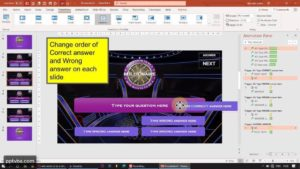## Create “Who Wants To Be A Millionaire” PowerPoint Game

Here, we will be using Microsoft PowerPoint to make an...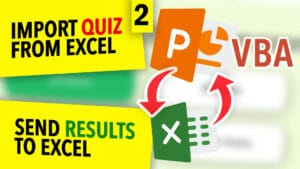## Populate A PowerPoint Quiz From Excel With VBA

Import Questions & Answers of a Microsoft PowerPoint Multiple-Choice Quiz...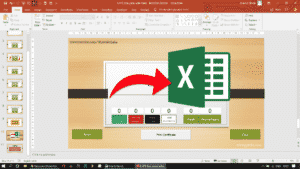## SAVE POWERPOINT QUIZ RESULTS TO EXCEL SHEET​

We will be exporting/sending the results of the PowerPoint Quiz...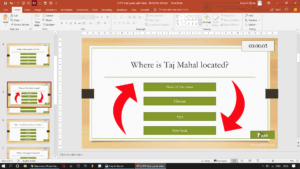## SHUFFLE QUESTIONS SLIDES & ANSWER ORDER RANDOMLY IN PPT QUIZ GAME​

We will be shuffling the order of the questions such...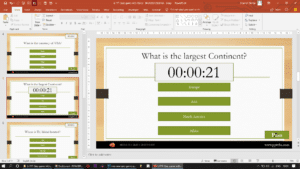## POWERPOINT QUIZ GAME WITH TIMER

As soon as the time limit is up, the student...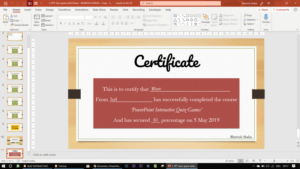## PRINT CERTIFICATE IN PPT QUIZ GAME

Collect student's name via an input field and use VBA...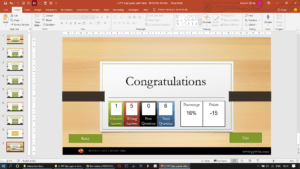## REPORT CARD AND PERCENTAGE – INTERACTIVE POWERPOINT QUIZ GAME

In this PowerPoint Quiz Game, we will calculate the number...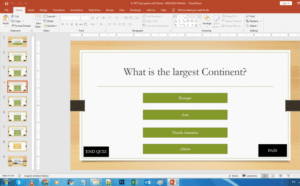## Interactive PowerPoint Quiz Game with Points and Scores

In this PowerPoint Quiz Module, we will be designing our...